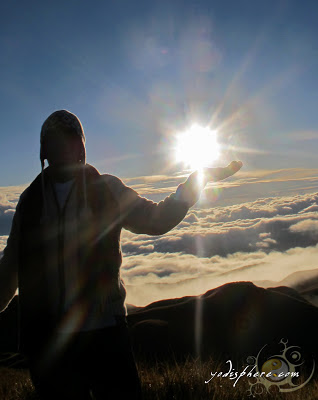## Tuesday, December 16, 2008

### The Beauty of Mathematics

1 x 8 + 1 = 9
12 x 8 + 2 = 98
123 x 8 + 3 = 987
1234 x 8 + 4 = 9876
12345 x 8 + 5 = 98765
123456 x 8 + 6 = 987654
1234567 x 8 + 7 = 9876543
12345678 x 8 + 8 = 98765432
123456789 x 8 + 9 = 987654321

1 x 9 + 2 = 11
12 x 9 + 3 = 111
123 x 9 + 4 = 1111
1234 x 9 + 5 = 11111
12345 x 9 + 6 = 111111
123456 x 9 + 7 = 1111111
1234567 x 9 + 8 = 11111111
12345678 x 9 + 9 = 111111111
123456789 x 9 +10= 1111111111

9 x 9 + 7 = 88
98 x 9 + 6 = 888
987 x 9 + 5 = 8888
9876 x 9 + 4 = 88888
98765 x 9 + 3 = 888888
987654 x 9 + 2 = 8888888
9876543 x 9 + 1 = 88888888
98765432 x 9 + 0 = 888888888

Brilliant, isn't it?

And look at this symmetry:

1 x 1 = 1
11 x 11 = 121
111 x 111 = 12321
1111 x 1111 = 1234321
11111 x 11111 = 123454321
111111 x 111111 = 12345654321
1111111 x 1111111 = 1234567654321
11111111 x 11111111 = 123456787654321
111111111 x 111111111 = 12345678987654321

Now, take a look at this...

101%
From a strictly mathematical viewpoint:

What Equals 100%?
What does it mean to give MORE than 100%?

Ever wonder about those people who say they are giving more than 100%?

We have all been in situations where someone wants you to
GIVE OVER 100%.

What equals 100% in life?

Here's a little mathematical formula that might help

If:

A B C D E F G H I J K L M N O P Q R S T U V W X Y Z

Is represented as:

1 2 3 4 5 6 7 8 9 10 11 12 13 14 15 16 17 18 19 20 21 22 23 24 25 26.

If:

H-A-R-D-W-O- R- K

8+1+18+4+23+ 15+18+11 = 98%

And:

K-N-O-W-L-E- D-G-E

11+14+15+23+ 12+5+4+7+ 5 = 96%

But:

A-T-T-I-T-U- D-E

1+20+20+9+20+ 21+4+5 = 100%

THEN, look how far the love of God will take you:

L-O-V-E-O-F- G-O-D

12+15+22+5+15+ 6+7+15+4 = 101%

Therefore, one can conclude with mathematical certainty that:

While Hard Work and Knowledge will get you close, and Attitude will
get you there, It's the Love of God that will put you over the top!

#### 1 comment:

1.prove: 1 + 1 = 0

hehehe.. may ganun talaga! may sagot mga mathematicians diyan!

Got Something to Say? Thoughts? Additional Information?Yodi Insigne is one of those delusional sorts who imagines himself a useful contributor to the greater blogosphere (Well, that's what he's trying to accomplish).

He started blogging for three reasons:

1. He always felt he has something important to say,
2. Books can make him cry, and cliff jumping can make him high,
3. He want to sleep at night.

He is a self-certified bookworm, travel junkie, shutterbug, movie freak, Mangyan hiker who sleeps a lot and think a lot. He got a little vice, which is black coffee and cashew nuts. He got colorblindness on yellow and green - and he freaking loves it!

### Mt. Pulag via Akiki-Ambangeg Trail » Odyssey to the Playground of the Gods